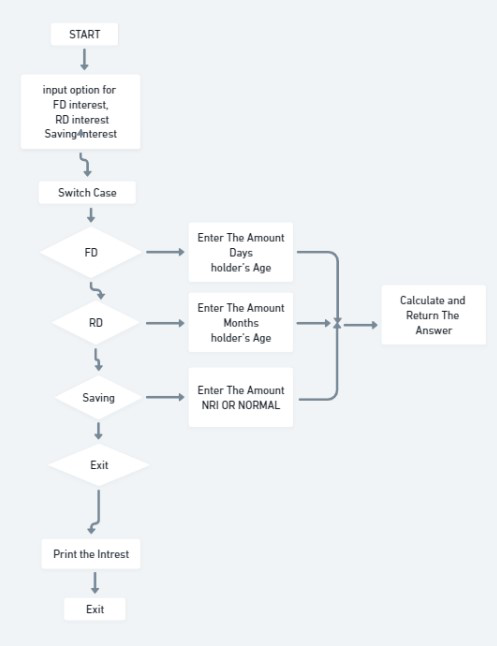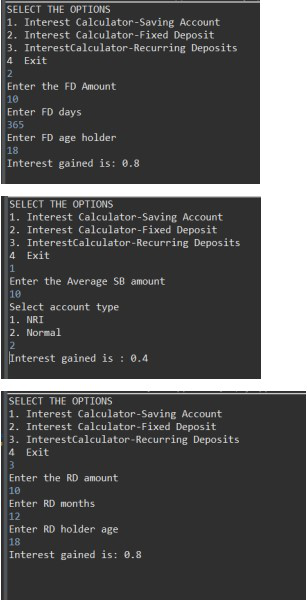# Java Program to Calculate Interest For FDs, RDs using Inheritance

• Last Updated : 23 May, 2022

Inheritance is an important pillar of OOP(Object-Oriented Programming). It is the mechanism in java by which one class is allowed to inherit the features(fields and methods) of another class. In this article, we will create an application to calculate interest for FDs, RDs based on certain conditions using inheritance. Create three classes in a single package. Create their member function. Create a Menu Driven Program.

### Steps

1. Create the Main Java File to take input of the choices on Interest Calculator like for Saving account, fixed deposit account, Recurring Deposits.
2. Create Another Class to calculate the Interest of fixed deposit. Calculate the FD Interest by Formula = FDAmount * FDinterestRate.
3. Also, Check If the Interest is Applicable in for normal Citizen Of the Senior Citizen. Create Another Class to calculate the Interest of Saving deposit. Calculate the saving account Interest by Formula = Amount * SavingAccountinterestRate. Also, check if it’s a Normal Account or an NRI Account.
4. Create Another Class to calculate the Interest of Recurring Deposits. Calculate the RD Interest by Formula = RDAmount * RDinterestRate.
5. Also, Check The Months and the holder’s age to Calculate the Interest.

### Flow DiagramAccount.Java

## Java

 `package` `College;`   `public` `abstract` `class` `Account {` `  `  `    ``double` `interestRate;` `    ``double` `amount;` `  `  `    ``abstract` `double` `calculateInterest(``double` `amount)` `        ``throws` `InvalidMonthsException, InvalidAgeException,` `               ``InvalidAmountException, InvalidDaysException;` `}`

IntrestCalculator.java

## Java

 `package` `College;`   `import` `java.util.Scanner;`   `public` `class` `InterestCalculator {` `    ``public` `static` `void` `main(String[] args)` `    ``{` `        ``// TODO code application logic here` `        ``Scanner sc = ``new` `Scanner(System.in);` `        ``System.out.println(` `            ``"SELECT THE OPTIONS "` `            ``+ ``"\n1."` `            ``+ " Interest Calculator-Saving` `              ``Account ``" + "` `\n2.``" + "` `Interest Calculator` `            ``- Fixed Deposit "` `            ``+ ``"\n3."` `            ``+ ``" InterestCalculator-Recurring Deposits"` `            ``+ ``"\n4 "` `            ``+ ``" Exit"``);` `        ``int` `choice = sc.nextInt();` `        ``switch` `(choice) {` `        ``case` `1``:` `            ``SBaccount sb = ``new` `SBaccount();` `            ``try` `{` `                ``System.out.println(` `                    ``"Enter the Average SB amount "``);` `                ``double` `amount = sc.nextDouble();` `                ``System.out.println(` `                    ``"Interest gained is : \$ "` `                    ``+ sb.calculateInterest(amount));` `            ``}` `            ``catch` `(InvalidAmountException e) {` `                ``System.out.println(` `                    ``"Exception : Invalid amount"``);` `            ``}` `            ``break``;` `        ``case` `2``:` `            ``try` `{` `                ``FDaccount fd = ``new` `FDaccount();` `                ``System.out.println(``"Enter the FD Amount"``);` `                ``double` `fAmount = sc.nextDouble();` `                ``System.out.println(` `                    ``"Interest gained is: \$ "` `                    ``+ fd.calculateInterest(fAmount));` `            ``}` `            ``catch` `(InvalidAgeException e) {` `                ``System.out.println(``"Invalid Age Entered"``);` `            ``}` `            ``catch` `(InvalidAmountException e) {` `                ``System.out.println(` `                    ``"Invalid Amount Entered"``);` `            ``}` `            ``catch` `(InvalidDaysException e) {` `                ``System.out.println(``"Invalid Days Entered"``);` `            ``}` `            ``break``;` `        ``case` `3``:` `            ``try` `{` `                ``RDaccount rd = ``new` `RDaccount();` `                ``System.out.println(``"Enter the RD amount"``);` `                ``double` `Ramount = sc.nextDouble();` `                ``System.out.println(` `                    ``"Interest gained is: \$ "` `                    ``+ rd.calculateInterest(Ramount));` `            ``}` `            ``catch` `(InvalidAgeException e) {` `                ``System.out.println(``"Invalid Age Entered"``);` `            ``}` `            ``catch` `(InvalidAmountException e) {` `                ``System.out.println(` `                    ``"Invalid Amount Entered"``);` `            ``}` `            ``catch` `(InvalidMonthsException e) {` `                ``System.out.println(``"Invalid Days Entered"``);` `            ``}` `            ``break``;` `        ``case` `4``:` `            ``System.out.println(` `                ``"DO YOU WANT TO CALCULATE AGAIN ????"` `                ``+ ``" "` `                ``+ ``"RUN AGAIN THE PROGRAM"``);` `        ``default``:` `            ``System.out.println(``"Wrong choice"``);` `        ``}` `    ``}` `}`

FDaAccount.java

## Java

 `package` `College;`   `import` `java.util.Scanner;`   `public` `class` `FDaccount ``extends` `Account {` `    ``double` `FDinterestRate;` `    ``double` `FDAmount;` `    ``int` `noOfDays;` `    ``int` `ageOfACHolder;` `    ``double` `General, SCitizen;` `    ``Scanner FDScanner = ``new` `Scanner(System.in);` `    ``@Override` `    ``double` `calculateInterest(``double` `amount)` `        ``throws` `InvalidAgeException, InvalidAmountException,` `               ``InvalidDaysException` `    ``{` `        ``this``.FDAmount = amount;` `        ``System.out.println(``"Enter FD days"``);` `        ``noOfDays = FDScanner.nextInt();` `        ``System.out.println(``"Enter FD age holder "``);` `        ``ageOfACHolder = FDScanner.nextInt();` `        ``if` `(amount < ``0``) {` `            ``throw` `new` `InvalidAmountException();` `        ``}` `        ``if` `(noOfDays < ``0``) {` `            ``throw` `new` `InvalidDaysException();` `        ``}` `        ``if` `(ageOfACHolder < ``0``) {` `            ``throw` `new` `InvalidAgeException();` `        ``}` `        ``if` `(amount < ``10000000``) {` `            ``if` `(noOfDays >= ``7` `&& noOfDays <= ``14``) {` `                ``General = ``0.0450``;` `                ``SCitizen = ``0.0500``;` `            ``}` `            ``else` `if` `(noOfDays >= ``15` `&& noOfDays <= ``29``) {` `                ``General = ``0.0470``;` `                ``SCitizen = ``0.0525``;` `            ``}` `            ``else` `if` `(noOfDays >= ``30` `&& noOfDays <= ``45``) {` `                ``General = ``0.0550``;` `                ``SCitizen = ``0.0600``;` `            ``}` `            ``else` `if` `(noOfDays >= ``45` `&& noOfDays <= ``60``) {` `                ``General = ``0.0700``;` `                ``SCitizen = ``0.0750``;` `            ``}` `            ``else` `if` `(noOfDays >= ``61` `&& noOfDays <= ``184``) {` `                ``General = ``0.0750``;` `                ``SCitizen = ``0.0800``;` `            ``}` `            ``else` `if` `(noOfDays >= ``185` `&& noOfDays <= ``365``) {` `                ``General = ``0.0800``;` `                ``SCitizen = ``0.0850``;` `            ``}` `            ``FDinterestRate` `                ``= (ageOfACHolder < ``50``) ? General : SCitizen;` `        ``}` `        ``else` `{` `            ``if` `(noOfDays >= ``7` `&& noOfDays <= ``14``) {` `                ``interestRate = ``0.065``;` `            ``}` `            ``else` `if` `(noOfDays >= ``15` `&& noOfDays <= ``29``) {` `                ``interestRate = ``0.0675``;` `            ``}` `            ``else` `if` `(noOfDays >= ``30` `&& noOfDays <= ``45``) {` `                ``interestRate = ``0.00675``;` `            ``}` `            ``else` `if` `(noOfDays >= ``45` `&& noOfDays <= ``60``) {` `                ``interestRate = ``0.080``;` `            ``}` `            ``else` `if` `(noOfDays >= ``61` `&& noOfDays <= ``184``) {` `                ``interestRate = ``0.0850``;` `            ``}` `            ``else` `if` `(noOfDays >= ``185` `&& noOfDays <= ``365``) {` `                ``interestRate = ``0.10``;` `            ``}` `        ``}` `        ``return` `FDAmount * FDinterestRate;` `    ``}` `}`

RDaccount.java

## Java

 `package` `College;`   `import` `java.util.Scanner;`   `public` `class` `RDaccount ``extends` `Account {` `    ``double` `RDInterestRate;` `    ``double` `RDamount;` `    ``int` `noOfMonths;` `    ``double` `monthlyAmount;` `    ``double` `General, SCitizen;` `    ``Scanner RDScanner = ``new` `Scanner(System.in);` `    ``@Override` `    ``double` `calculateInterest(``double` `Ramount)` `        ``throws` `InvalidMonthsException,` `               ``InvalidAmountException, InvalidAgeException` `    ``{` `        ``this``.RDamount = Ramount;` `        ``System.out.println(``"Enter RD months"``);` `        ``noOfMonths = RDScanner.nextInt();` `        ``System.out.println(``"Enter RD holder age"``);` `        ``int` `age = RDScanner.nextInt();` `        ``if` `(RDamount < ``0``) {` `            ``throw` `new` `InvalidAmountException();` `        ``}` `        ``if` `(noOfMonths < ``0``) {` `            ``throw` `new` `InvalidMonthsException();` `        ``}` `        ``if` `(age < ``0``) {` `            ``throw` `new` `InvalidAgeException();` `        ``}` `        ``if` `(noOfMonths >= ``0` `&& noOfMonths <= ``6``) {` `            ``General = .``0750``;` `            ``SCitizen = ``0.080``;` `        ``}` `        ``else` `if` `(noOfMonths >= ``7` `&& noOfMonths <= ``9``) {` `            ``General = .``0775``;` `            ``SCitizen = ``0.0825``;` `        ``}` `        ``else` `if` `(noOfMonths >= ``10` `&& noOfMonths <= ``12``) {` `            ``General = .``0800``;` `            ``SCitizen = ``0.0850``;` `        ``}` `        ``else` `if` `(noOfMonths >= ``13` `&& noOfMonths <= ``15``) {` `            ``General = .``0825``;` `            ``SCitizen = ``0.0875``;` `        ``}` `        ``else` `if` `(noOfMonths >= ``16` `&& noOfMonths <= ``18``) {` `            ``General = .``0850``;` `            ``SCitizen = ``0.0900``;` `        ``}` `        ``else` `if` `(noOfMonths >= ``22``) {` `            ``General = .``0875``;` `            ``SCitizen = ``0.0925``;` `        ``}` `        ``RDInterestRate = (age < ``50``) ? General : SCitizen;` `        ``return` `RDamount * RDInterestRate;` `    ``}` `}`

SBaccount.Java

## Java

 `package` `College;`   `import` `java.util.Scanner;`   `class` `SBaccount ``extends` `Account {` `    ``double` `SBamount, SbInterestRate, interest;` `    ``Scanner SBScanner = ``new` `Scanner(System.in);` `    ``@Override` `    ``double` `calculateInterest(``double` `amount)` `        ``throws` `InvalidAmountException` `    ``{` `        ``this``.SBamount = amount;` `        ``if` `(SBamount < ``0``) {` `            ``throw` `new` `InvalidAmountException();` `        ``}` `        ``System.out.println(` `            ``"Select account type \n1. NRI \n2. Normal "``);` `        ``int` `accountChoice = SBScanner.nextInt();` `        ``switch` `(accountChoice) {` `        ``case` `1``:` `            ``SbInterestRate = .``06``;` `            ``break``;` `        ``case` `2``:` `            ``SbInterestRate = .``04``;` `            ``break``;` `        ``default``:` `            ``System.out.println(` `                ``"Please choose right account again"``);` `        ``}` `        ``return` `amount * SbInterestRate;` `    ``}` `}`

### OutputMy Personal Notes arrow_drop_up
Recommended Articles
Page :